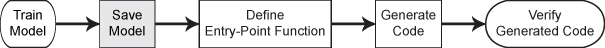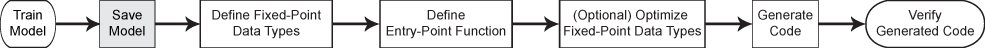# saveLearnerForCoder

Save model object in file for code generation

Since R2019b

## Syntax

``saveLearnerForCoder(Mdl,filename)``

## Description

To generate C/C++ code for the object functions of machine learning models (including `predict`, `random`, `knnsearch`, `rangesearch`, `isanomaly`, and incremental learning functions), use `saveLearnerForCoder`, `loadLearnerForCoder`, and `codegen` (MATLAB Coder). After training a machine learning model, save the model by using `saveLearnerForCoder`. Define an entry-point function that loads the model by using `loadLearnerForCoder` and calls an object function. Then use `codegen` or the MATLAB® Coder™ app to generate C/C++ code. Generating C/C++ code requires MATLAB Coder.

This flow chart shows the code generation workflow for the object functions of machine learning models. Use `saveLearnerForCoder` for the highlighted step.Fixed-point C/C++ code generation requires an additional step that defines the fixed-point data types of the variables required for prediction. Create a fixed-point data type structure by using the data type function generated by `generateLearnerDataTypeFcn`, and use the structure as an input argument of `loadLearnerForCoder` in an entry-point function. Generating fixed-point C/C++ code requires MATLAB Coder and Fixed-Point Designer™.

This flow chart shows the fixed-point code generation workflow for the `predict` function of a machine learning model. Use `saveLearnerForCoder` for the highlighted step.example

````saveLearnerForCoder(Mdl,filename)` prepares a model (`Mdl`) for code generation and saves it in the MATLAB formatted binary file (MAT-file) named `filename`. You can pass `filename` to `loadLearnerForCoder` to reconstruct the model object from the `filename` file.```

## Examples

collapse all

After training a machine learning model, save the model by using `saveLearnerForCoder`. Define an entry-point function that loads the model by using `loadLearnerForCoder` and calls the `predict` function of the trained model. Then use `codegen` (MATLAB Coder) to generate C/C++ code.

This example briefly explains the code generation workflow for the prediction of machine learning models at the command line. For more details, see Code Generation for Prediction of Machine Learning Model at Command Line. You can also generate code using the MATLAB Coder app. See Code Generation for Prediction of Machine Learning Model Using MATLAB Coder App for details. To learn about the code generation for finding nearest neighbors using a nearest neighbor searcher model, see Code Generation for Nearest Neighbor Searcher.

Train Model

Load Fisher's iris data set. Remove all observed setosa irises data so that `X` and `Y` contain data for two classes only.

```load fisheriris inds = ~strcmp(species,'setosa'); X = meas(inds,:); Y = species(inds);```

Train a support vector machine (SVM) classification model using the processed data set.

`Mdl = fitcsvm(X,Y);`

`Mdl` is a `ClassificationSVM` object, which is a linear SVM model. The predictor coefficients in a linear SVM model provide enough information to predict labels for new observations. Removing the support vectors reduces memory usage in the generated code. Remove the support vectors from the linear SVM model by using the `discardSupportVectors` function.

`Mdl = discardSupportVectors(Mdl);`

Save Model

Save the SVM classification model to the file `SVMIris.mat` by using `saveLearnerForCoder`.

`saveLearnerForCoder(Mdl,'SVMIris');`

Define Entry-Point Function

Define an entry-point function named `classifyIris` that does the following:

• Accept iris flower measurements with columns corresponding to `meas`, and return predicted labels.

• Load a trained SVM classification model.

• Predict labels using the loaded classification model for the iris flower measurements.

```function label = classifyIris(X) %#codegen %CLASSIFYIRIS Classify iris species using SVM Model % CLASSIFYIRIS classifies the iris flower measurements in X using the SVM % model in the file SVMIris.mat, and then returns class labels in label. Mdl = loadLearnerForCoder('SVMIris'); label = predict(Mdl,X); end ```

Add the `%#codegen` compiler directive (or pragma) to the entry-point function after the function signature to indicate that you intend to generate code for the MATLAB algorithm. Adding this directive instructs the MATLAB Code Analyzer to help you diagnose and fix violations that would result in errors during code generation.

Note: If you click the button located in the upper-right section of this example and open this example in MATLAB®, then MATLAB® opens the example folder. This folder includes the entry-point function file.

Generate Code

Generate code for the entry-point function using `codegen` (MATLAB Coder). Because C and C++ are statically typed languages, you must determine the properties of all variables in the entry-point function at compile time. Pass `X` as the value of the `-args` option to specify that the generated code must accept an input that has the same data type and array size as the training data `X`. If the number of observations is unknown at compile time, you can also specify the input as variable-size by using `coder.typeof` (MATLAB Coder). For details, see Specify Variable-Size Arguments for Code Generation and Specify Properties of Entry-Point Function Inputs (MATLAB Coder).

`codegen classifyIris -args {X}`
```Code generation successful. ```

`codegen` generates the MEX function `classifyIris_mex` with a platform-dependent extension.

Verify Generated Code

Compare the labels classified using `predict`, `classifyIris`, and `classifyIris_mex`.

```label1 = predict(Mdl,X); label2 = classifyIris(X); label3 = classifyIris_mex(X); verify_label = isequal(label1,label2,label3)```
```verify_label = logical 1 ```

`isequal` returns logical 1 (true), which means all the inputs are equal. The labels classified all three ways are the same.

After training a machine learning model, save the model using `saveLearnerForCoder`. For fixed-point code generation, specify the fixed-point data types of the variables required for prediction by using the data type function generated by `generateLearnerDataTypeFcn`. Then, define an entry-point function that loads the model by using both `loadLearnerForCoder` and the specified fixed-point data types, and calls the `predict` function of the model. Use `codegen` (MATLAB Coder) to generate fixed-point C/C++ code for the entry-point function, and then verify the generated code.

Before generating code using `codegen`, you can use `buildInstrumentedMex` (Fixed-Point Designer) and `showInstrumentationResults` (Fixed-Point Designer) to optimize the fixed-point data types to improve the performance of the fixed-point code. Record minimum and maximum values of named and internal variables for prediction by using `buildInstrumentedMex`. View the instrumentation results using `showInstrumentationResults`; then, based on the results, tune the fixed-point data type properties of the variables. For details regarding this optional step, see Fixed-Point Code Generation for Prediction of SVM.

Train Model

Load the `ionosphere` data set and train a binary SVM classification model.

```load ionosphere Mdl = fitcsvm(X,Y,'KernelFunction','gaussian');```

`Mdl` is a `ClassificationSVM` model.

Save Model

Save the SVM classification model to the file `myMdl.mat` by using `saveLearnerForCoder`.

`saveLearnerForCoder(Mdl,'myMdl');`

Define Fixed-Point Data Types

Use `generateLearnerDataTypeFcn` to generate a function that defines the fixed-point data types of the variables required for prediction of the SVM model.

`generateLearnerDataTypeFcn('myMdl',X)`

`generateLearnerDataTypeFcn` generates the `myMdl_datatype` function.

Create a structure `T` that defines the fixed-point data types by using `myMdl_datatype`.

`T = myMdl_datatype('Fixed')`
```T = struct with fields: XDataType: [0x0 embedded.fi] ScoreDataType: [0x0 embedded.fi] InnerProductDataType: [0x0 embedded.fi] ```

The structure `T` includes the fields for the named and internal variables required to run the `predict` function. Each field contains a fixed-point object, returned by `fi` (Fixed-Point Designer). The fixed-point object specifies fixed-point data type properties, such as word length and fraction length. For example, display the fixed-point data type properties of the predictor data.

`T.XDataType`
```ans = [] DataTypeMode: Fixed-point: binary point scaling Signedness: Signed WordLength: 16 FractionLength: 14 RoundingMethod: Floor OverflowAction: Wrap ProductMode: FullPrecision MaxProductWordLength: 128 SumMode: FullPrecision MaxSumWordLength: 128 ```

Define Entry-Point Function

Define an entry-point function named `myFixedPointPredict` that does the following:

• Accept the predictor data `X` and the fixed-point data type structure `T`.

• Load a fixed-point version of a trained SVM classification model by using both `loadLearnerForCoder` and the structure `T`.

• Predict labels and scores using the loaded model.

```function [label,score] = myFixedPointPredict(X,T) %#codegen Mdl = loadLearnerForCoder('myMdl','DataType',T); [label,score] = predict(Mdl,X); end ```

Note: If you click the button located in the upper-right section of this example and open the example in MATLAB®, then MATLAB opens the example folder. This folder includes the entry-point function file.

Generate Code

The `XDataType` field of the structure `T` specifies the fixed-point data type of the predictor data. Convert `X` to the type specified in `T.XDataType` by using the `cast` (Fixed-Point Designer) function.

`X_fx = cast(X,'like',T.XDataType);`

Generate code for the entry-point function using `codegen`. Specify `X_fx` and constant folded `T` as input arguments of the entry-point function.

`codegen myFixedPointPredict -args {X_fx,coder.Constant(T)}`
```Code generation successful. ```

`codegen` generates the MEX function `myFixedPointPredict_mex` with a platform-dependent extension.

Verify Generated Code

Pass predictor data to `predict` and `myFixedPointPredict_mex` to compare the outputs.

```[labels,scores] = predict(Mdl,X); [labels_fx,scores_fx] = myFixedPointPredict_mex(X_fx,T);```

Compare the outputs from `predict` and `myFixedPointPredict_mex`.

`verify_labels = isequal(labels,labels_fx)`
```verify_labels = logical 1 ```

`isequal` returns logical 1 (true), which means `labels` and `labels_fx` are equal.

If you are not satisfied with the comparison results and want to improve the precision of the generated code, you can tune the fixed-point data types and regenerate the code. For details, see Tips in `generateLearnerDataTypeFcn`, Data Type Function, and Fixed-Point Code Generation for Prediction of SVM.

## Input Arguments

collapse all

Machine learning model, specified as a full or compact model object, as given in the following tables of supported models. The tables also show whether each model supports fixed-point code generation.

File name, specified as a character vector or string scalar.

If the `filename` file exists, then `saveLearnerForCoder` overwrites the file.

The extension of the `filename` file must be `.mat`. If `filename` has no extension, then `saveLearnerForCoder` appends `.mat`.

If `filename` does not include a full path, then `saveLearnerForCoder` saves the file to the current folder.

Example: `'SVMMdl'`

Data Types: `char` | `string`

## Tips

• Before saving the model using the `saveLearnerForCoder` function, you can remove support vectors from a linear SVM model or an ECOC model with linear SVM learners by using the `discardSupportVectors` function. The predictor coefficients in a linear SVM model provide enough information to predict labels and responses for new observations, and removing the support vectors reduces memory usage in the generated code.

• If `Mdl` is a linear SVM model, and the model has both predictor coefficients and support vectors, then you can remove the support vectors from the model by using the `discardSupportVectors` function (for classification) or the `discardSupportVectors` function (for regression). By default, an SVM model with a linear kernel includes both predictor coefficients and support vectors.

• If `Mdl` is an ECOC model with linear SVM learners, and the learners have both predictor coefficients and support vectors, then you can remove the support vectors from the learners by using the `discardSupportVectors` function. The default `SaveSupportVectors` value of linear SVM learners is `false`. Therefore, by default, an ECOC model does not include support vectors for the learners.

## Algorithms

`saveLearnerForCoder` prepares a machine learning model (`Mdl`) for code generation. The function removes some unnecessary properties.

• For a model that has a corresponding compact model, the `saveLearnerForCoder` function applies the appropriate `compact` function to the model before saving it.

• For a model that does not have a corresponding compact model, such as `ClassificationKNN`, `ClassificationKernel`, `ClassificationLinear`, `RegressionKernel`, `RegressionLinear`, `ExhaustiveSearcher`, `KDTreeSearcher`, and `IsolationForest`, the `saveLearnerForCoder` function removes properties such as hyperparameter optimization properties, training solver information, and others.

`loadLearnerForCoder` loads the model saved by `saveLearnerForCoder`.

## Version History

Introduced in R2019b